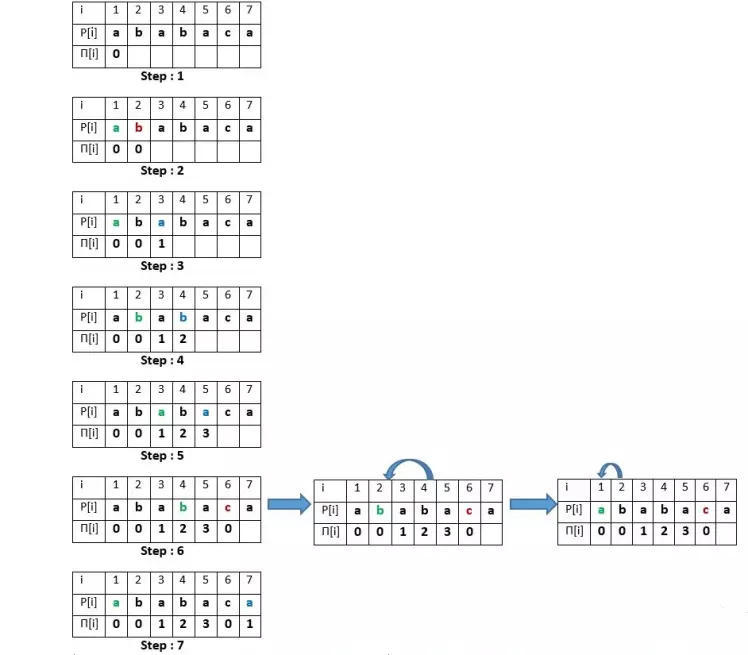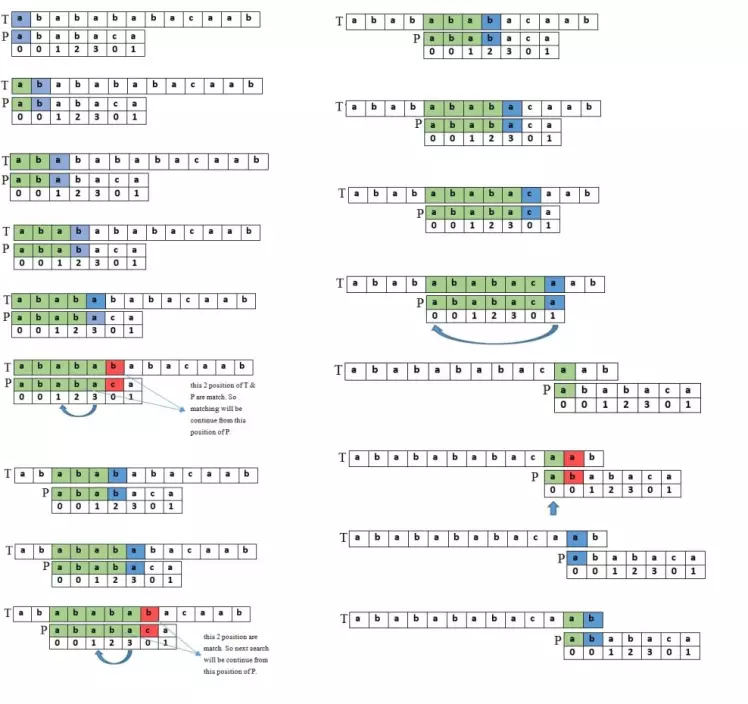# Prefix function - Knuth-Morris-Pratt Algorithm

## Introduction

KMP is the most popular substring search algorithm in linear time. This algorithm is used for finding linear time solutions of various string-related problems such as searching substring in a string, the number of unique substrings in a string, pattern matching problem, etc.

## Algorithm

The main component of the KMP algorithm is the prefix function. It is defined as for a string s of length n, π[i] is the length of the longest proper prefix of the substring s[0…i], which is also a suffix of this substring where the proper prefix of a string is a prefix that is not equal to the string itself. π[i] is the ith element of an n-length array π. By definition, π=0.

π[i]= max

k=0...i

k : s[0…k−1]=s[i−(k−1)…i],

where s[0…k−1] is the proper prefix and s[i−(k−1)…i] is the suffix of length k of the string s.

e.g  for string "ababaca", prefix function array π is [0,0,1,2,3,0,1]source

## Brute force approach

It takes O(n3) in the worst case.

## Optimized approach:

• At first, compute the prefix values π[i] in a loop by iterating from i=1 to i=n−1 (π is assigned with 0).
• To calculate the current value π[i], set the variable j denoting the length of the best suffix for i−1. Initially, j=π[i−1].
• Test if the suffix of substring s[0....i] of length j+1 is also a prefix of the substring s[0....i] by comparing s[j] and s[i]. If they are equal, we assign π[i]=j+1 (the values of the prefix function can only increase by at most one); otherwise, reduce j to π[j−1] ( greatest k<j, for which the prefix property holds is π[j−1]) and repeat this step.
• If length j=0 is reached and still don't have a match, then assign π[i]=0 and go to the next index i+1

## Complexity analysis

The KMP algorithm takes O(n) time in the worst case and space complexity is also O(n) for using an extra array of length n.

## Application

Searching a substring in a string

Searching a pattern P in string S using the ∏ function is just like making the ∏ function. If we get any difference in S and P, we can jump on a character in S with the help of ∏ function because we already equaled the suffix and prefix. Go through the below example for understanding how prefix function is used for pattern matching.source

Output

## FAQs

1. What is the application of the KMP algorithm?
KMP algorithm is used for searching a substring in a string, the number of unique substrings in a string, pattern matching problem.

2. What is the alternative algorithm of the Knuth-Morris-Pratt algorithm?
Robin-Karp algorithm is an alternative to the KMP algorithm.

3. What is the proper prefix of a string?
The proper prefix of a string is a substring that is not equal to the length of the string itself.

## Key Takeaways

This article covered the KMP algorithm. We have learned about the algorithm to apply it for solving various string-related problems.

Now, you can practice and solve a wide range of string-related coding questions commonly asked in interviews in CodeStudio. Along with coding questions, we can also find the interview experience of scholars working in renowned product-based companies here.

Happy learning!